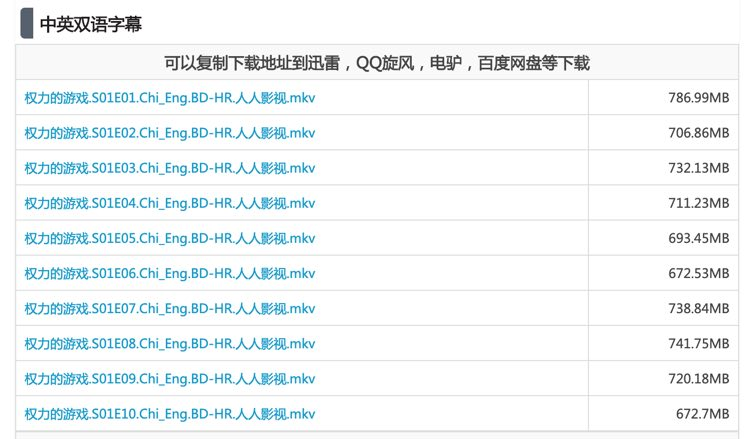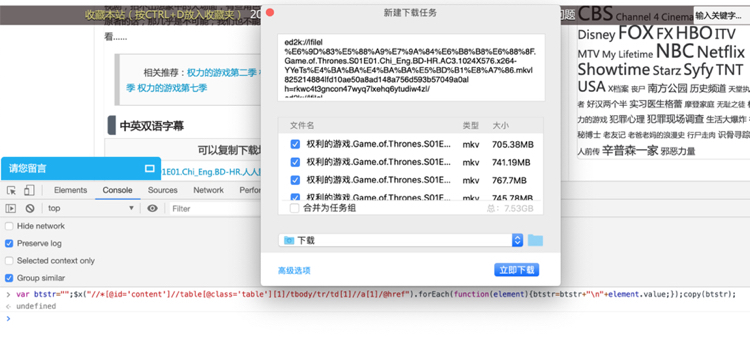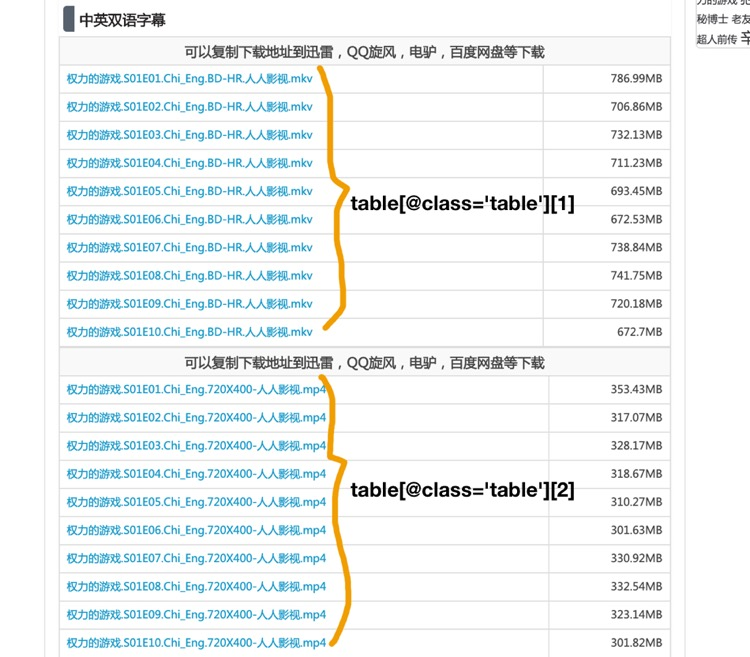### 步骤一

``var btstr="";\$x("//*[@id='content']//table[@class='table']/tbody/tr/td//a/@href").forEach(function(element){btstr=btstr+"\n"+element.value;});copy(btstr);``### 小技巧

``````var btstr = "";
\$x("//*[@id='content']//table[@class='table']/tbody/tr/td//a/@href").forEach(function(element) {
btstr = btstr + "\n" + element.value;
});
copy(btstr);``````

``````var btstr = "";
\$x("//*[@id='content']//table[@class='table']/tbody/tr/td//a/@href").forEach(function(element) {
btstr = btstr + "\n" + element.value;
});
copy(btstr);``````